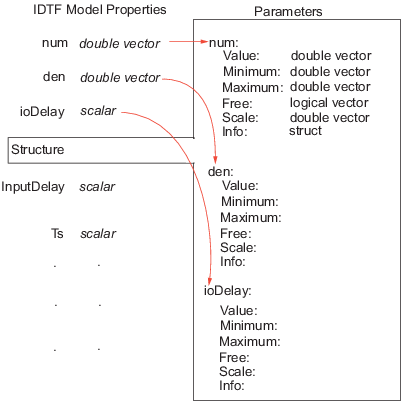## Imposing Constraints on Model Parameter Values

All identified linear (IDLTI) models, except `idfrd`, contain a `Structure` property. The `Structure` property contains the adjustable entities (parameters) of the model. Each parameter has attributes such as value, minimum/maximum bounds, and free/fixed status that allow you to constrain them to desired values or a range of values during estimation. You use the `Structure` property to impose constraints on the values of various model parameters.

The `Structure` property contains the essential parameters that define the structure of a given model:

• For identified transfer functions, includes the numerator, denominator, and delay parameters

• For polynomial models, includes the list of active polynomials

• For state-space models, includes the list of state-space matrices

For information about other model types, see the model reference pages.

For example, the following example constructs an `idtf` model, specifying values for the `Numerator` and `Denominator` parameters:

```num = [1 2]; den = [1 2 2]; sys = idtf(num,den)```

You can update the value of the `Numerator` and `Denominator` properties after you create the object as follows:

```new_den = [1 1 10]; sys.Denominator = new_den; ```

To fix the denominator to the value you specified (treat its coefficients as fixed parameters), use the `Structure` property of the object as follows:

```sys.Structure.Denominator.Value = new_den; sys.Structure.Denominator.Free = false(1,3); ```

For a transfer function model, the `Numerator`, `Denominator`, and `IODelay` model properties are simply pointers to the `Value` attribute of the corresponding parameter in the `Structure` property.Similar relationships exist for other model structures. For example, the `A` property of a state-space model contains the double value of the state matrix. It is an alias to the `A` parameter value stored in `Structure.A.Value`.

## SupportGet trial now